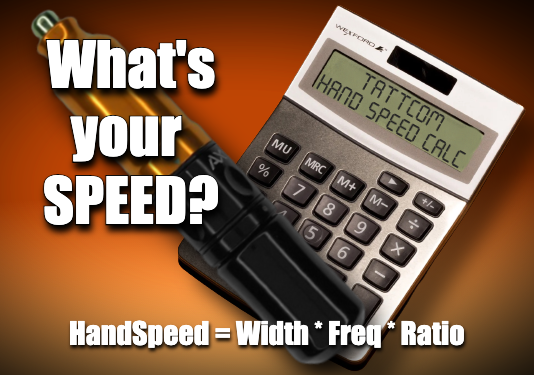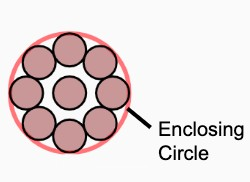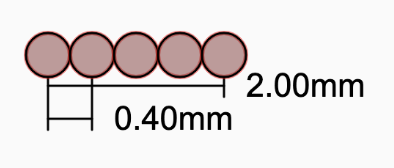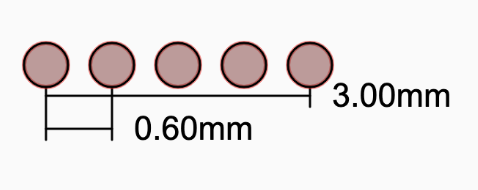# Tattoo Hand Speed Calculator## Why it matters

Tattoo lining requires consistency. The punctures made by the tattooing needle(s) need to be in perfect harmony with the reciprocation rate of the tattoo machine, as well as in sync with the artists hand speed.

If one of these variables is not in sync with the other the needle punctures can be placed too closely together resulting in overlap which can tear the skin. On the other hand, if the neelde punctures are too far apart then the desired density and saturation is not achieved

With the right balance of hand speed and machine speed one can find the “sweet spot” where the ink seems to be deposited effortlessly into the skin.

Use the calculator to find the right hand speed for your setup today, to get the perfect machine speed every time check out the ESCx Power Supply.

## How to use

This calculator will allow you to visualize the interdependent relationships of each variable in the formula and see how they impact each other and the calculated hand and machine speeds.

### Hand Speed = Grouping Width * Frequency * Ratio

Here is the formula, you can find detailed descriptions of each of the variables below.

## (W) Grouping WidthThis is the width of the grouping. For round groupings is is the enclosing circle diameter, and for flat mags it is the width of the stacked needles.(ratio 1:1)(ratio 1.5:1)

This is the advance ratio of the needle grouping in relation to the machine reciprocation speed. If we were to move the needle grouping by one time (1x) the enclosing circle diameter per cycle of the machine we will have a 1:1 ratio.

## (F) Frequency

This is the reciprocation frequency of the needle grouping (hz/cps). In other words it is the number of times the needle reciprocates over a given period of time, usually 1 second. You can calculate frequency by dividing the number of cycles over the time taken to complete. For example if a tattoo machines needle reciprocates 10 times in one second it's frequency is 10/1 or 10hz.  It is important to remember this number must be a constant, if it changes the hand speed needs to change as well.